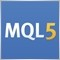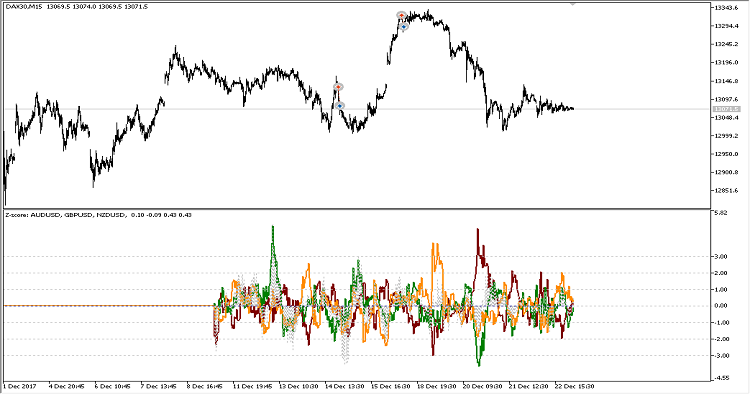Indicators: Cointegration148099

The indicator calculates and displays a linear relationship between two or more financial symbols.Author: Maxim Dmitrievsky38

Author: Maxim Dmitrievsky

Hi @Maxim Dmitrievsky,

Great Indicator. I just have a few clarifications.

1. If I have a mix pairs of XXXUSD and USDXXX, does it convert all USDXXX to XXXUSD?

2. Im assumming it is using Johansen test to test multiple relationships and getting the Beta to form the Linear cointegration relationship and have a basis for Z-score probability? How does it work?

Thanks!

regards,

Francis6

Author: Maxim Dmitrievsky6

961011:
thanks706

franzzzz:

1. If I have a mix pairs of XXXUSD and USDXXX, does it convert all USDXXX to XXXUSD?

2. Im assumming it is using Johansen test to test multiple relationships and getting the Beta to form the Linear cointegration relationship and have a basis for Z-score probability? How does it work?

1. no, it doesn't convert anything to the currency of your deposit, so it will not work properly for USDCHF, EURGBP, USDCAD, etc

2. no, it's using simple linear regression between multiple assets and then divide all charts by Standard Deviation to make it look like flat channel15

Can you clarify what the 0 line signifies?  It's little confusing to me how the lines oscillate between positive (above the 0) and negative (below the 0) when standard deviations cannot be negative.  Thanks!6

So, it does not give any clue whether the paired assets are cointegrated or not, doesn't it?
It just use simple regression to find the beta and coefficient, and get the error (or spread), and then divide the spread by its standard deviation to get the signal. Am I right?

Thanks Maxim.29537

bqFX:

So, it does not give any clue whether the paired assets are cointegrated or not, doesn't it?
It just use simple regression to find the beta and coefficient, and get the error (or spread), and then divide the spread by its standard deviation to get the signal. Am I right?

Thanks Maxim.

yes, without tests on cointegration